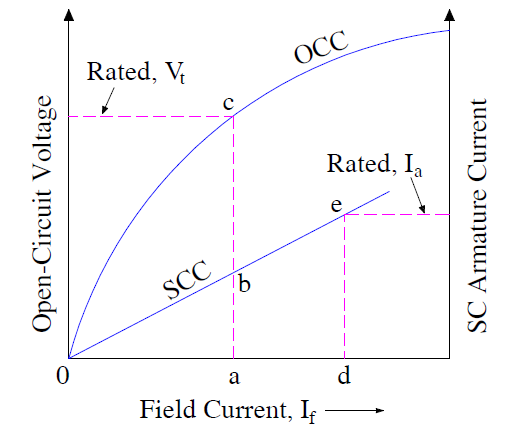# Short Circuit Ratio-Definition, Calculation and Significance

### Definition

Short Circuit Ratio, or SCR, is the ratio of field current required to generate rated voltage under open circuit condition, to the field current required to circulate the rated armature current under three phase short circuit condition. It is an important quantity of synchronous machine which helps in estimation of operating characteristics. It is also useful for obtaining the size of machine for a given rating and type.

The value of Short Circuit Ratio varies from 0.5 to 0.8 for Turbo Generator, 1 to 1.4 for Hydro generator and 0.4 to 0.5 for synchronous condenser.

Calculation

Short Circuit Ratio can easily be obtained from the Open Circuit (OCC) and Short Circuit Characteristics (SCC) of Synchronous machine. Figure below shows the OCC and SCC.From the above figure and definition,

SCR = If for rated OC voltage / If for rated SC current

= (oa / od) ……(1)

But triangle oab and ode are similar triangles, therefore

oa/od = ab/de

From (1),

SCR = (ab/de) …..(2)

Direct axis reactance Xd of synchronous machine is defined as the ratio of open circuit voltage for a given field current, to the armature short circuit current for the same filed current. Therefore, direct axis reactance Xd for field current oa is given as

Xd = (ac/ab) Ω

The per unit (p.u) value of direct axis reactance Xd is obtained by dividing it by base impedance.

p.u value of Xd = (ac/ab) / Base Impedance

But,

Base Impedance = Vrated / Irated

From the Open Circuit and Short Circuit Characteristics,

Vrated = ac Volt

Irated = de Amp.

Therefore, base impedance = (ac/de) Ω

P.u value of Xd = (ac/ab)x(de/ac)

= (de/ab)

From (2),

Xd in p.u = 1/SCR

SCR = 1/ Xd in per unit

Thus, short circuit ratio is equal to the reciprocal of per unit value of direct axis synchronous reactance. The more the value of Xd, the lesser will be the short circuit ratio.

### Significance of Short Circuit Ratio

Value of short circuit ratio, or SCR, affects both the size and operating characteristics of synchronous machine. Significance of SCR can be better understood by considering the following cases:

#### Low SCR:

Low value of SCR simply means greater value of Xd. The voltage regulation is greatly affected by direct axis reactance. More the value of Xd, the poor will be the voltage regulation. This means that, the terminal voltage will widely vary with variation in load. In order to main constant terminal voltage, the field current needs to be varied widely and therefore the load on the excitation system will increase.

Greater value of direct axis reactance Xd also affects the stability of synchronous machine. The synchronizing power of synchronous machine is defined as the degree by which the machine has a tendency to get synchronized with the infinite Grid just after experiencing disturbance. Low value of synchronizing power means, the machine will have fewer tendencies to get synchronized again after recovering from disturbance.

Synchronizing Power is inversely proportional to reactance Xd, lower value of short circuit ratio results in lower stability limit.

The only advantage of lower SCR is the lower value of armature current during three phase short circuit.

#### High SCR:

High value of short circuit ratio gives improved voltage regulation. Thus the terminal voltage is practically constant during the load variation. It also results in better stability limit due to increased value of synchronizing power.

But the disadvantage of high value of SCR is increased armature current during three phase short circuit and increased machine size.

#### Size and Cost of Machine

SCR greatly affects the size and hence the cost of machine. To better understand the effect of Short Circuit Ratio on the size of machine, let us assume that the air gap length is doubled. The mutual inductance Md, in terms of direct axis (d-axis) synchronous reactance Xd can be written as,

Md α 1/d-axis reactance

Doubling the air gap length doubles the reluctance. Therefore, the magnetic flux linkage becomes half. Thus the mutual inductance reduces to half of its original value. To main same open circuit terminal voltage, filed current must be doubled.

The short circuit current Isc is given as

Isc = Ef/Xd

= MdωIf / √3ωLd

= MdIf / √3Ld

where Ld is direct axis inductance.

When air gap length is doubled, mutual inductance Md and self inductance Ld are equally affected. Therefore, the field current required to circulate the rated armature current remains same. It is thus observed that when air gap length is doubled,

• Field current is doubled for maintain same open circuit voltage
• Field current remains same for circulating rated armature current

As per the definition of SCR, doubling the air gap length doubles the short circuit ratio. With double the air gap, the field current required to generate the same open circuit terminal voltage is doubled. This requires greater cross-section of field winding and hence greater field copper.  This increased amount of copper field winding can only be wound if the field pole size is increased. Subsequently, the machine diameter increases and hence overall size, weight and cost of machine increases.

### 2 thoughts on “Short Circuit Ratio-Definition, Calculation and Significance”

1.SUMIT SOURAVA MOHANTY says:

Woow great , the way you have tried to explain is very convincing…
Good good Keep it up………… upload more lectures..

1.admin says:

Thank you! Please share if you like it.

This site uses Akismet to reduce spam. Learn how your comment data is processed.